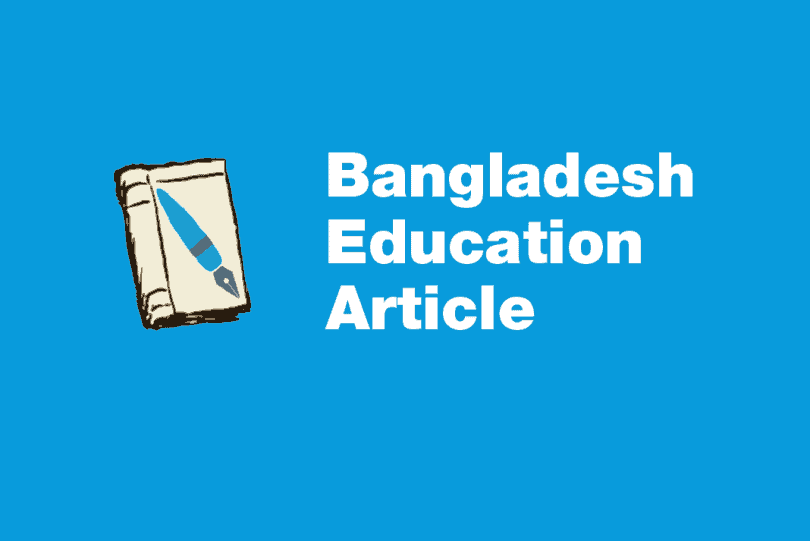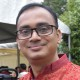Primary

# List of subjectwise competencies in Mathematics for primary education of BangladeshBangladesh Education ArticleWritten by

There are total 37 subjectwise competencies found for mathematics subject. National Curriculum and Textbook Board (NCTB) has made those subjectwise competencies. The total 37 competencies for mathematics are given below.

1. To count any kind of numerous objects.
2. To count up to crore numbers.
3. To identify 0 to 9 numerical digits.
4. To read up to crore numbers.
5. To write up to crore numbers.
6. To identify roman numerical digits up to twelve.
7. To estimate place value which are used in numbers (up to crore).
8. To arrange number (up to crore) in order.
9. To read, write and use of sequential numbers (up to twenty).
10. To add two or more than two numbers (carrying digit and not).

11. To subtract one number from another (carrying digit and not).
12. To solve mathematical problem by using addition and subtraction procedure.
13. To multiply one number by another (multiplicand would be utmost four digit in number and multiplier at least three digit in number).
14. To divide one number by another (dividend would be utmost four digit in number and divisor at least three digit in number).
15. To solve mathematical problem by using addition, subtraction, multiplication and divisor procedure(in any stage of procedure greater than four digit number not to be used)
16. To recognized Bangladeshi coin and taka and use in daily transition.
17. To know unitary methods and to solve simple mathematical problem.
18. To simplify integer number attached equation.
19. To obtain the concept of average and to solve related simple problem.
20. To obtain the concept of H.C.F. and L.C.M. and use this to solve related simple problem.
21. To solve problem by using mathematical notation.
22. To obtain the concept of common fraction and identify different types of fraction and use it (denominator utmost two digit number).
23. To add, subtract, multiply and division of common fraction (denominator would be two digit number).
24. To solve simple problem based on addition, subtraction, multiplication and division of common fraction (denominator of fraction would be utmost two digit number).
25. To obtain the concept and use of decimal fraction.
26. To solve simple problem based on addition, subtraction, multiplication and division of decimal fraction (denominator of fraction would be utmost two digit number).
27. To obtain the concept of percentage and use it in problem solving.
28. Capable to know the different measurement units of length, weight, volume and area and use it.
29. To know the time measurement unit and use it.
30. To keep the record of income-expenditure and use it.
31. To collect data from different source of environment and arrange it.
32. To obtain the concept graph and express different data by using graph.
33. To recognized different geometrical shape of different environmental elements and express it by letters.
34. To classify, draw and express the plane geometrical shapes (triangle, quadrilateral and circle).
35. To know the concept of point, line and surface.
36. To know the usage of calculator and use it in calculation.
37. To solve simple population related problems by using mathematical knowledge.

Source: National Curriculum and Textbook Board, Bangladesh. Translated by Rifat Afroze.

### About the author